关于函数f(x)和函数f(-x)：

1、函数f(x)和函数f(-x)是两个函数， 因为函数f(-x)的对应关系是f(-())， 而函数f(x)的对应关系是f()，f(-())比f()，多了一个“对自变量x求反”运算；

1）举例说，对数函数logax和对数函数loga(-x)，对数函数loga(-x)对自变量x有一个求反的运算；

2）当x是负数时，-x就是一个正数；

3）这样对数函数loga(-x)的定义域就是x＜0，-x是个正数；

4）而对数函数logax的定义域是x＞0，x是个正数；

5）所以函数loga(-x)的图像在3、4象限，而函数logax的图像在1、2象限；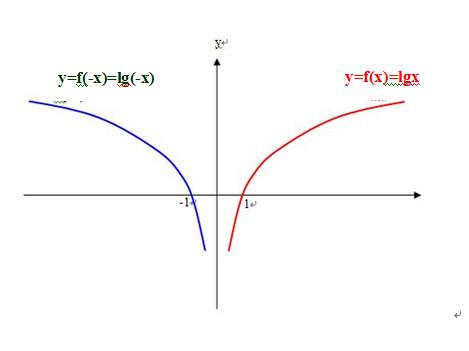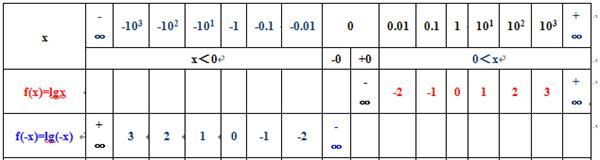2、函数f(x)和函数f(-x)是两个相关函数， 因为函数f(-x)的对应关系是f(-()) 而函数f(x)的对应关系是f(  )f(-())f()都有共同的f(  )

1）举例说，对数函数loga(x)和对数函数loga(-x)，都是对数函数，都有loga( )

2）因为负数没有对数，或者说真数只能是正数，所以loga(x)的定义域是x＞0；

3）因为负数没有对数，或者说真数只能是正数，所以loga(-x)的定义域是x＜0,，-x是正数；

4）所以对数函数loga(-x)的图像在3、4象限，而对数函数logax的图像在1、2象限；

3、对于一个任意的有确切定义的已知 函数f(x)， 一定有一个函数 f(-x)与之对应存在；

1）举例说，已知对数函数y=lg(x)在0到正的无穷大区间上；

2）就一定有对数函数y=lg(-x)在0到负的无穷大区间上；.

.

3）举例说，已知幂函数y=(x)^2，在(-∞，+∞)上；

4）就一定有幂函数；y=(-x)^2，在(-∞，+∞)上；

5）因为y=(x)^2=(-x)^2，所以幂函数y=(x)^2是个偶函数，幂函数y=(-x)^2也是个偶函数；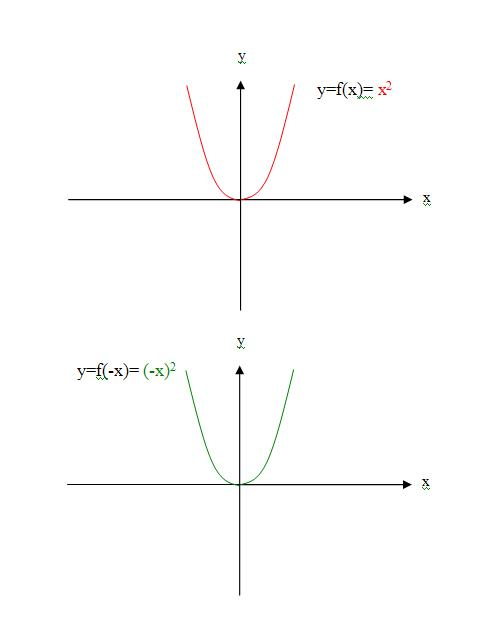6）举例说，已知幂函数y=(x)^3，在(-∞，+∞)上；

7）就一定有幂函数；y=(-x)^3，在(-∞，+∞)上；

8）因为y=(x)^3=-(-x)^3，所以幂函数y=(x)^3是个奇函数，幂函数y=(-x)^3也是个奇函数；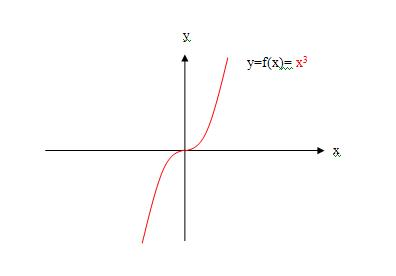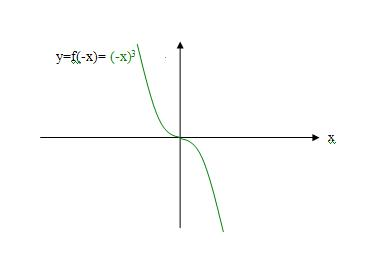4、由于有以上函数基础知识存在， 所以出题的人， 才会出主楼这样的题目， 他只需要告诉已知函数f(x)的情况，做题的人自然会求得函数f(-x)、f(x)-f(-x)；

5、由于wanggq和寒湘子，没有上述函数基础知识，对函数f(x)、f(-x)的关系乱作解释，给主楼题目解出了无数多个正确的解所以无知是错误和谎言之源！！！或者说错误和谎言欺骗的是无知者！！！或者说无知是错误和谎言得以传播的动力 ！！！

6、我们看到，无知的wanggq时不时的搬出“正统大学教材”一些零碎章节的内容截图，却能堂而皇之的把它歪曲成“错误和谎言”，让人们亲身体会到错误与谎言往往是打着科学、技术的幌子欺骗无知者，捞取不义之财！

7、wanggq是怎么堂而皇之的把正确的知识歪曲成“错误和谎言”的呢？

1）在研究偶函数图像以y轴为对称这个性质时，教材中有这样一段叙述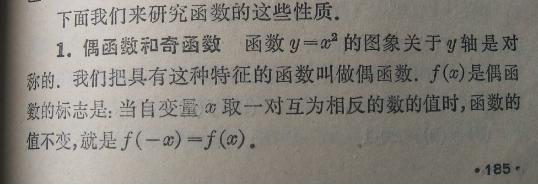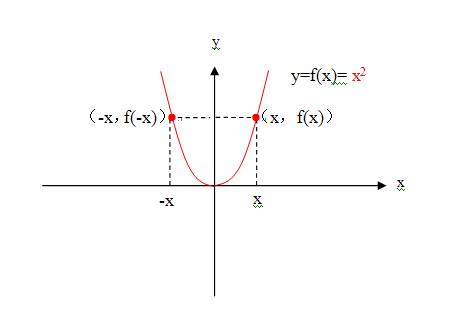2）根据教材的论述，我们同样可以得到：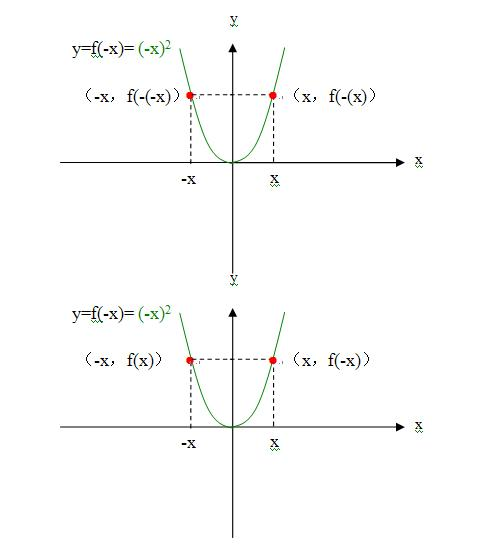3）这样我们知道函数y=x^2是偶函数，那么函数y=(-x)^2也是偶函数，也就是说，当函数f(x)是偶函数时，一定有函数f(-x)也是偶函数，它们的图像以y轴为对称，以y轴对折时完全重合，函数f(-x)自变量-x对应的函数值f(x)与函数f(x)自变量x对应的函数值f(x)重合；如下图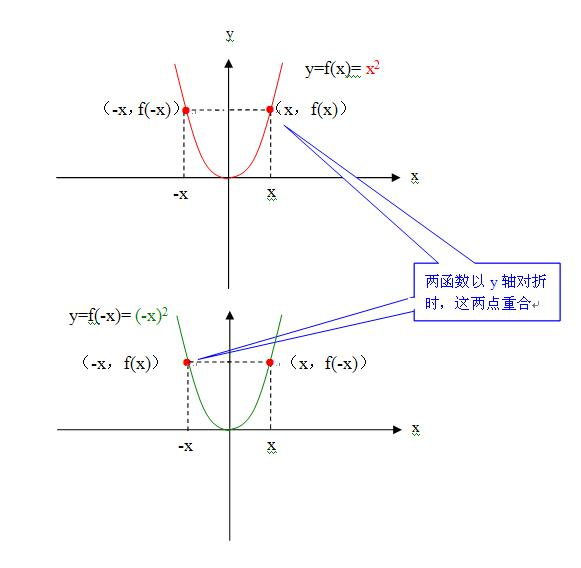3）这样我们得到这样一个结论，偶函数时，函数f(x)与函数f(-x)两个都是偶函数，其图像以y轴为对称，以y轴对折时完全重合；

4）如果已知函数f(x)不是偶函数，它的图像，与f(x)相关的函数f(-x)的图像也是以y轴为对称，以y轴对折时，完全重合；5）wanggq是怎么堂而皇之的把正确的知识歪曲成“错误和谎言”的呢？如截图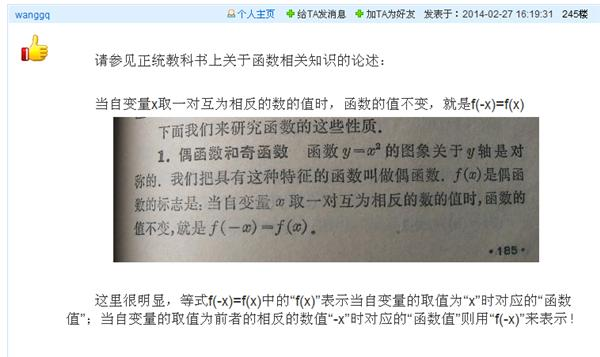wanggq说，“请参见正统教科书上关于函数相关知识的论述：……当自变量x取一对互为相反的数的值时，函数的值不变，就是f(-x)=f(x) ，……这里很明显，等式f(-x)=f(x)中的“f(x)”表示当自变量的取值为“x”时对应的“函数值”；当自变量的取值为前者的相反的数值“-x”时对应的“函数值”则用“f(-x)”来表示！ wanggq以此为据，认定主楼题目“f(x)在0到正无穷大是增函数，f(1)=0，求（f(x)-f(-x)）/x<0时x的范围”中，f(-x)是已知函数f(x)图像上“自变量取相反数值“-x”时对应的“函数值”！ wanggq以此为据，认定主楼题目“f(x)在0到正无穷大是增函数，f(1)=0，求（f(x)-f(-x)）/x<0时x的范围”中，已知函数f(x)的定义域必然是（-∞，+∞）……